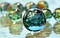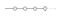# Testing RxJS Observables with Marble DiagramsMarbles Diagrams will help us testing RxJS Obaservables (📷 from WallpaperUp)

Testing RxJS might be a hard task, specially when we have a complex pipeline with lots of time assertions. In this article we’ll explore how can we make these tests easier to reason about using Marble Diagrams, but first:

# What are Marble Diagrams?

Marble Diagrams are visual representation for events emitted over the time. For example, this is the diagram corresponding to an emission from 0 to 3 over the time:You can take a look at this diagram at rxviz.com, a very usefull website for generaating these diagrams.

Now that we have this basic time interval we can transform the data, for example multiplying each number by two:

This means that there exists a function called numTwoTimes that will map each of the values of the source observable to its multiplication by two. This is the implementation:

# Testing RxJS without Marbles Diagrams

In order to test our function, we have to send it values through an observable, so we’ll create an observable that emits 4 values over the time, and then subscribe to the observable and check that the emitted values are right:

This example might look simple at first, but we are not testing the time of the emissions (that would be very hard), despite it takes 3 seconds to execute the test.

# Testing with marble diagrams

To test with marble diagrams, we need to represent this diagram using ASCII characters, so the previous example becomes:

`-a–b–c-|numTwoTimes-x-y-z-|values = { a: 1, b: 2, c: 3, x: 2, y: 4, z: 6 }`

From this snippet, we can extract several things:

• : Means a frame of time, that is by default 10ms.
• character: It’s a single emitted value, it’s like a variable name with its value defined at the object.
• : It represent the completation of the observable.

So with this ASCII marble diagram, we can rewrite our tests as the following:

This way we are asserting not only the values, but also the timing of the emissions. Also, we fix the time of the execution, as RxJS’s uses virtual time, so tests are actually synchronous!

# Conclusion

• It’s easier to test Observable behavior, specially the time of the emitted values.
• It’s easier to read, as you only have to look at the diagram instead of reading the code.
• It’s faster, as it uses virtual time to simulate delays, intervals, etc…

You can take a look for more avanced examples and concepts (like error handling or grouping emissions) at this repo:

## More From Medium

#### Also tagged JavaScript

Apr 9 · 5 min read